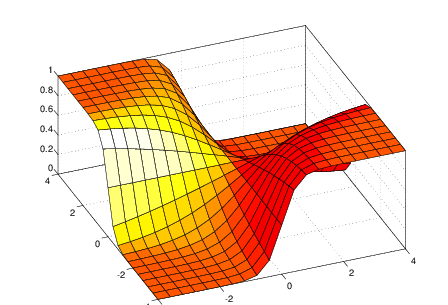# Logistic Regression in depth

Source: Deep Learning on Medium# Logistic Regression in depth

Logistic regression

We use logistic regression for basic as well as complex classifications in our data or we can say that we use this algorithm to assign our observations from the data to a discrete set of classes.

This algorithm uses the logistic sigmoid function to return a probability value.

(but wait what’s a sigmoid function?)

Sigmoid function

This function maps any real value into another value between the scale of 0 and 1. In machine learning, we use this function to map predictions to probabilities. The value where X=0 is exactly 0.5, so in this sample we can consider 0.5 as a threshold value for determining the classes (i.e. 1 or 0). If the output is greater than 0.5, we can classify it as class-1(Y=1) or if it’s less than 0.5 we say it belongs to class-0(Y=0).

Now let us see how we can use logistic regression for classification purposes

If you know linear regression (if not read it from the link at the bottom) and we can say that logistic regression is more of a generalized form of linear regression, here instead of outputting the weighted sum of inputs directly, we pass it through a function that can map any real value between 0 and 1.

If we take the weighted sum of inputs as the output as we do in linear regression, the value can be more than 1 but we are not looking for values that are not between 0 and 1. We can say, that this the reason linear regression can’t be used for classification purposes.

Let’s look at some assumptions of logistic regression

• For logistic regression, we require quite a large sample size.
• But only unique and meaningful variables should be included.
• The independent variables are linearly related to the log odds.
• The model should have little or no multicollinearity.
• Binary logistic regression requires the dependent variable to be binary.

Decision boundary: it’s the boundary that separates discrete data values e.g. in the above example 0.5 can be said as the decision boundary.

Vectorization

Vectorization is a technique where we avoid using loops because it approves efficiency and speed. Let’s see with an example. We have 2 arrays both consists of 1 million elements each and if we multiply both the array element-wise & sum the elements in resulting array by (1) for loop, (2) vectorization, and then we will compare the time difference.